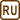## Cooling Water for a Bath

### Task number: 1793

There are 220 litres of water at a temperature of 55 °C in a bathtub. How much water at a temperature of 14 °C should we add to the water in the bathtub, so that the resultant water temperature is 40 °C. The heat transfer between the water and its environment is not considered.

Assume the density of the water is 1000 kg/m3.

• #### Hint

To solve the problem, we are going to use the calorimetric formula, which states that the heat supplied by a warmer object is equal to the heat received by a cooler object (the law of conservation of energy).

• #### Notation

 Vh = 220 l volume of hot water th = 55 °C temperature of hot water tc = 14 °C temperature of cold water tf = 40 °C required final water temperature ρ = 1000 kgm−3 density of water Vc = ? desired volume of cold water
• #### Analysis

In the solution of this problem we are going to use the calorimetry formula, which states that the sum of the heats supplied by warmer bodies to cooler bodies equals the sum of the heats received by the cooler bodies from the warmer bodies. Considering given information, we can compute the heat, which the hot water needs to give up to cool its temperature down until it is equal to the required temperature. From this heat we can determine the mass of cold water.

• #### Solution

The heat $$Q_1$$supplied by the hot water to the cold water:

$Q_1 = c_w m_h (t_h - t_f)= c_w V_h \varrho (t_h - t_f) \,.$

The same amount of heat is received by the cold water. For received heat $$Q_2$$ it holds that:

$Q_2 = c_w m_c (t_f - t_c) = c_w V_c \varrho (t_f - t_c)\,.$

According to the calorimetry formula the heats are equal.

$Q_1 = Q_2$

$c_w V_h \varrho (t_h - t_f) = c_w V_c \varrho (t_f - t_c)$

From the last equation we can determine the volume of cold water:

$V_h (t_h - t_f) = V_c (t_f - t_c)$

$V_c = V_h \frac { t_h - t_f}{t_f - t_c}$

Finally, we can substitute given values into the equation (since the calculation requires the differences of the temperatures, it is irrelevant whether we use the Celsius or Kelvin scale). If we substitute the volume of hot water in litres, computed volume of cold water is also in litres.

$V_c = 220 \cdot \frac { 55 - 40}{40 - 14}\,\mathrm l = 127 \,\mathrm l$

• #### Answer

The volume of cold water necessary to cool the water to the required temperature is 127 litres.